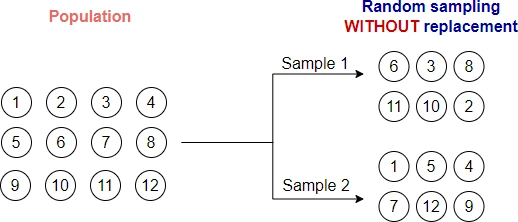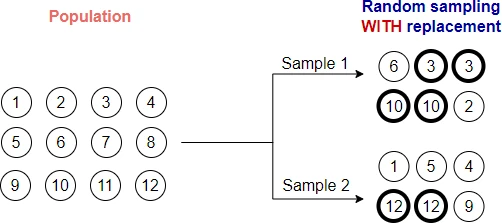# Understanding random sampling with and without replacement (with python code)

Statistics and machine learning rely heavily on random sampling (or simple random sampling). Basically, random sampling refers to the selection of observations from a large dataset (population) at random, where each observation has an equal chance of being chosen.

For example, in a bag of 100 balls, if we select any 10 balls and every ball has an equal chance of selection, then it is called a random sample.

Simple Random sampling can be divided into sampling without replacement and sampling with replacement based on the method of selection.

## Sampling without replacement

In sampling without replacement method, the samples are selected randomly from the original dataset (population) without any replacement. That is if one sample is selected, it will not be selected again.For example, in a bag of 10 balls, we can have two random samples of 5 balls. Every ball has an equal chance of selection.

Let’s perform random sampling without replacement using `random.sample()` function in Python

``````from random import sample

# list of 10 balls
bag = list(range(1, 11))
# output
[1, 2, 3, 4, 5, 6, 7, 8, 9, 10]

# select random sample of size 5 (without replacement)
sample(bag, 5)
# output
[2, 5, 8, 6, 4]
``````

In the above example, you can see sample of size 5 drawn randomly without replacement from a bag of 10 balls.

## Sampling with replacement

In the sampling with replacement method, the samples are selected randomly from the original dataset (population) with possible replacement. That is if one sample is selected, it may be selected again.For example, in bag of 10 balls, we can select one ball randomly and make a record of it. Then put that back again in the bag and select second ball. Repeat the process until required size of sample. In this sampling, there is chance that same ball can be selected multiple times.

Let’s perform random sampling without replacement using `random.choices()` function in Python

``````from random import choices

# bag of 10 balls
bag = list(range(1, 11))
# output
[1, 2, 3, 4, 5, 6, 7, 8, 9, 10]

# select random sample of size 5 (with replacement)
choices(bag, k=5)
# output
[10, 8, 6, 10, 8]
``````

In the above example, you can see a sample of size 5 drawn randomly with replacement (some balls are repetitive) from a bag of 10 balls.

## Enhance your skills with courses on machine learning

If you have any questions, comments or recommendations, please email me at reneshbe@gmail.com